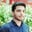Related Tags

python programming
communitycreator

# How to use count() method of string and bytes in PythonSalman Yousaf

### Overview

The count() method helps find the number of occurrences of subsequence in the specified range containing the start and end index. The start and end parameters are optional and deduced as slice notation.

### Syntax

str.count(sub, start = 0, end = len(string))


### Parameters

• sub: Represents the subsequence that is to be searched.
• start: The index for starting the search. The index of the first character is 0. The default value of the start parameter is 0.
• end: Represents the ending index of the search. Its value is the last index.

### Return value

This method returns the number of occurrences of subsequence in the defined binary data or bytes-like object.

### Example

In the code snippet below, we will use this method to search sub-strings from the parent string:

str = "01011101 01110101"
# COUNT NUMBER OF 0's
sub = '0'
print ("str.count('0') : ", str.count(sub))
# COUNT NUMBER OF 1's from 1 to 8
# IT WILL RETURN COUNT FROM 1 TO LESS THAN 8
sub = '1'
print ("str.count('str', 1, 8) : ", str.count(sub,1,8))
Using the count function with a string

### Explanation

• Line 4: We count for a total number of zeros (sub-string) in the parent string str. We can use a sub-string to argue the count() method.
• Line 8: We count the number of 1’s from the string str by using count() from 1st position to 8th.

### Syntax

bytes.count(sub[, start[, end]])


### Parameters

• Subsequence sub ranges between start and end.
• The argument to count() method must be a byte object like “abc” string or a “c” string literal or a number between 0 and 255.
• start and end arguments are optional.

#### Return value

This method returns the number of occurrences.

### Syntax

bytearray.count(sub[, start[, end]])


### Parameters

• Subsequence sub ranges between start and end.
• The argument to count() must be a bytearray object, like “abc” string or a “c” string literal or a number between 0 and 255.
• start and end arguments are optional.

### Return value

This method returns the number of occurrences.

### Example

The code snippet below demonstrates the use of the count() method from bytes and bytearray classes:

# Create a bytes object and a bytearray.
# bytes type object
data = bytes(b"aabbbccc")
# bytearray type object
arr = bytearray(b"aabbcccc")
# The count method works on both.
print("Count of c: ", data.count(b"c"))
print("Count of c: ", arr.count(b"c"))
Using the count function with a bytes and a bytearray

### Explanation

• Line 3: We initialize and define a bytes type instance.
• Line 5: We initialize and define a bytearray type instance.
• Lines 7 and 8: We use the count() function to check the number of occurrences of the specified argument value i.e., the character c.

RELATED TAGS

python programming
communitycreator

CONTRIBUTORSalman Yousaf
RELATED COURSES

View all Courses

Keep Exploring

Learn in-demand tech skills in half the time